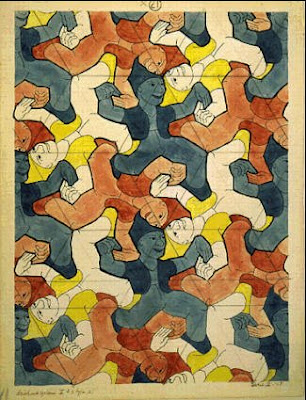## Haag Hexagon and its generalization to a Haag Polygon

Construction: Construct equilateral △ABC. 1) Draw a circle with centre A and arbitrary radius AD. 2) Construct a circle with centre B and radius BD. Label as P the other intersection of the two circles centred at A and B. 3) Draw a circle with centre C and radius CP. Label as E and F respectively the other intersections of circle C with circles B and A. Then ∠DAF = ∠DBE = ∠ECF = 120° and ADBECF is a Haag hexagon. The construction and properties of a Haag hexagon is mentioned in the notes of the famous Dutch artist M.C. Escher (see Schattschneider, 1990, p. 90).

Reference
Schattschneider, D. (1990). M.C. Escher: Visions of Symmetry. New York: W.H. Freeman & Co.

#### .sketch_canvas { border: medium solid lightgray; display: inline-block; } A Construction of the Haag hexagon (and more)

Challenges
1) Can you explain why (prove) ∠DAF = ∠DBE = ∠ECF = 120° from the above construction?
2) Can you explain why (prove) the main diagonals AE, BF and DC of the Haag hexagon ADBECF are concurrent?

Haag Tiling
One can create a tiling with the Haag hexagon, by starting with a basic tiling of the plane with equilateral triangles, and then covering the plane by rotating the Haag hexagon by 120° and/or 240° around each vertex of the equilateral triangular grid as shown in the dynamic sketch above - click on the 'Link to Haag Tiling' button on the bottom right of the sketch.

It is not clear whether Escher actually used the Haag tiling to create some of his art, but it is strongly suggestive that some of his work such as the ‘running men’ shown below displays the same rotational symmetry as the Haag tiling.Haag Polygon
One way of generalizing the Haag hexagon is by applying the Haag ‘circle’ construction mentioned above to a general triangle as well as to other polygons such as quadrilaterals, pentagons, hexagons, etc. to create a ‘Haag polygon’. Though the general Haag hexagon created from a general triangle does not tile and its main diagonals are not concurrent, it still has the Haag property as shown in the dynamic sketch above, that irrespective of the position of point D, ∠DAF = 2∠BAC, ∠DBE = 2∠ABC and ∠ECF = 2∠BCA. Click on the 'Link to General Triangle' button on the bottom right of the sketch to view the case for a general triangle.

Challenges & Further Investigation
1) Can you explain why (prove that) the angle relationship above is valid for a general Haag hexagon, and for a Haag polygon?
2) Drag the red vertices until the circumcentre O of △ABC coincides with P. What do you notice about the sides of the hexagon ADBFCE in this case? Can you prove your observations?
3) For the hexagon in 2), can you produce a tiling?

Conjecturing: Haag Octagon & Haag Dodecagon
Click on the relevant "Link' buttons in the above sketch to navigate to dynamic sketches for a Haag octagon AEBFCGDH and a Haag dodecagon AGBHCIDJEKFL, respectively created from a parallelogram ABCD and a hexagon ABCDEF with opposite sides parallel. Drag the points as indicated. What do you notice? Can you make some conjectures?

Challenge
State your conjectures related to the above two sketches and explain why (prove that) they are true.

Read my paper An Investigation of Some Properties of the General Haag Polygon (pdf, 553 KB, Mathematics in School, 43(3), May 2014, pp. 15-18).

Created by Michael de Villiers, 27 October 2013; updated to WebSketchpad, 26 January 2022.